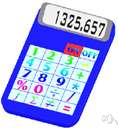expected value

(redirected from Expectation value)
Also found in: Thesaurus, Financial, Acronyms, Encyclopedia.

ex·pect·ed value

(ĭk-spĕk′tĭd)
n.
1. A quantity expressing a typical or average value of a random variable.
2. The sum (for discrete variables) or integral (for continuous variables) of the product of a random variable with its probability density function, over its range of values.

expected value

n
(Statistics) statistics the sum or integral of all possible values of a random variable, or any given function of it, multiplied by the respective probabilities of the values of the variable. Symbol: E(X). E(X) is the mean of the distribution; E(X–c) = E(X)–c where c is a constant. Also called: mathematical expectation
ThesaurusAntonymsRelated WordsSynonymsLegend:
 Noun 1expected value - the sum of the values of a random variable divided by the number of valuesstatistics - a branch of applied mathematics concerned with the collection and interpretation of quantitative data and the use of probability theory to estimate population parametersmean, mean value - an average of n numbers computed by adding some function of the numbers and dividing by some function of n
References in periodicals archive ?
The expectation value E (E = b + au) represents the maximum possible credibility between A and B.
Another classical treatment of quantum gravity comes from Roger Penrose with the Gravitization of Quantum Mechanics  where he posits that gravity connects not to the expectation value, but rather directly to each superposed quantum state.
The charge density at each site in the unit cell is calculated as the expectation value of the charge density operator [[??].sup.[dagger].sub.i](t)[[??].sub.i](t) over the many-body ground state.
However, the two-point correlation function obtained by this way is not the expectation value, <0 [absolute value of ([phi][phi])] 0>(x), which we expect for the energy-momentum tensor.
The customer total consumption and the service expectation value are very low so this kind of customers value is low.
In order to turn it into a practical scheme for calculating the transverse dynamic spin susceptibility, two further problems must still be addressed, however: First, the expectation value involving products of four field operators on the right-hand side must be expressed in terms of [[chi].sup.+-](r, r'; t - t') or other, known quantities.
Taking into account decline of monitoring quality followed by increasing distance between interested target and node, along with the deflected angle between the interested target and current sensing direction of node ([??][T.sub.k][s.sub.i]C as shown in Figure 2), the coverage expectation value of node to interested target is defined.
The expectation value of [x.sup.2] in the density matrix [[??].sub.u](x,f) can be determined from the definition of the expectation values as
Let [??] = ([s.sub.[alpha]], [[g.sup.L.sub.A], [g.sup.U.sub.A]]) be the interval linguistic variable, the expectation value of interval grey linguistic variable [??] is defined as follows:
The soil expectation value results reflect the same trend found in the net present value analysis, at slightly higher values per acre.
In composite models an [a.sub.LR]-interaction must contain the factor [nu]/[LAMBDA] relative to the SU(2)[.sub.L] X U(1) invariant interactions, where v is the vacuum expectation value of the SM Higgs boson and [LAMBDA] is the compositness scale.

Site: Follow: Share:
Open / Close